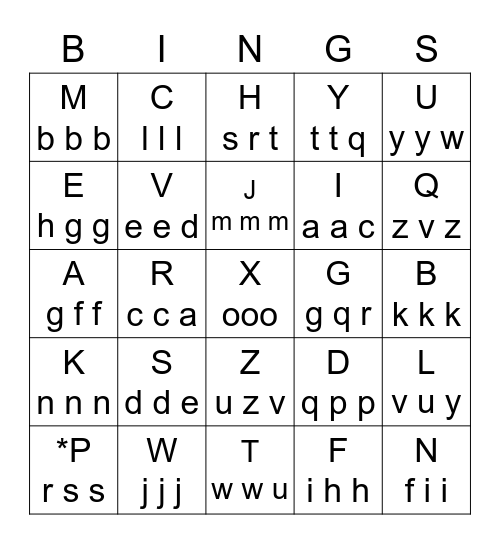# Ps Cl Gr Ly Ra VdThis bingo card has a free space and 24 words: S d d e, W j j j, J m m m, *P r s s, U y y w, M b b b, E h g g, C l l l, G g q r, Z u z v, I a a c, A g f f, D q p p, L v u y, V e e d, F i h h, B k k k, H s r t, Q z v z, R c c a, N f i i, K n n n, Y t t q and T w w u.

## Play Online